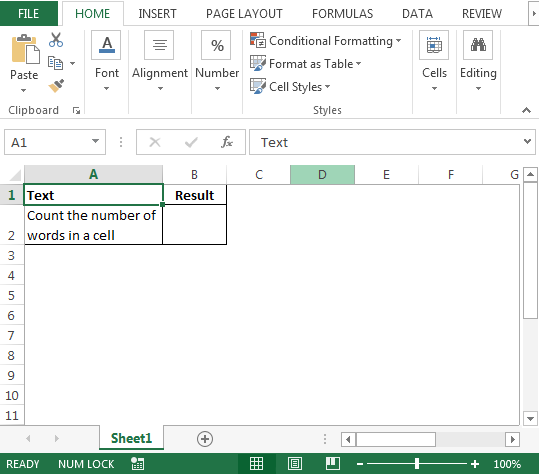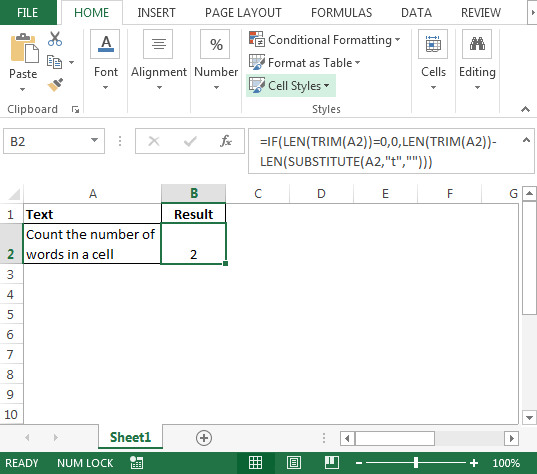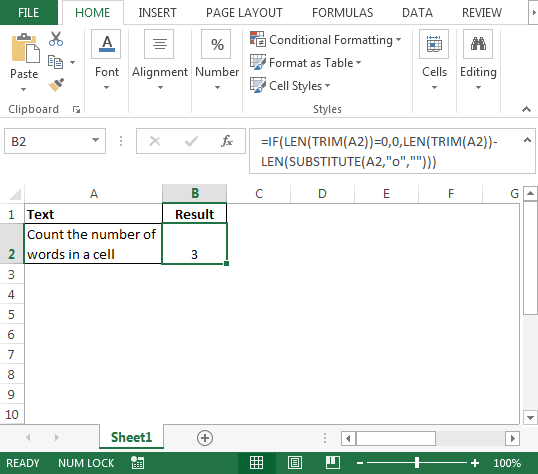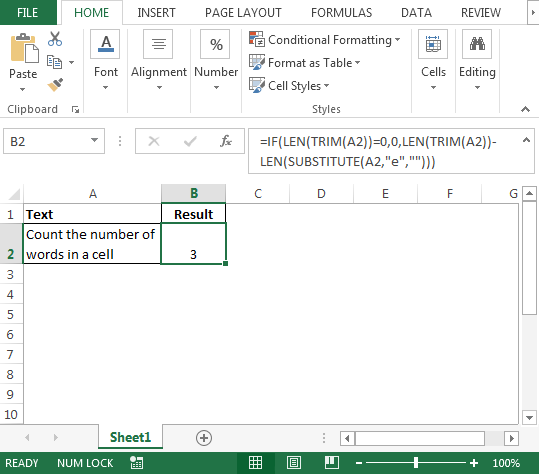# Count the number of words in a cell in Microsoft Excel

In case you have a requirement to count the number of particular word is repeated in a cell then you must read this article. In this article, we will use combination of IF, LEN, TRIM & SUBSTITUTE functions to achieve the output.

Example: If the text is “Count the number of words in a cell” & we want to find out how many times character “t” is repeated or say any character, then we can simply use the combination of above functions  in order to get the result.

Following is the snapshot of sample data:• In cell B2, the formula will be
• =IF(LEN(TRIM(A2))=0,0,LEN(TRIM(A2))-LEN(SUBSTITUTE(A2,"t","")))• This means character “t” has been repeated 2 times in the above string

In case the character is changed from “t” to “o” then we need to make slight adjustment in the formula i.e. replace “t” with “o”

• In cell B2, the formula will be
• =IF(LEN(TRIM(A2))=0,0,LEN(TRIM(A2))-LEN(SUBSTITUTE(A2,"o","")))• This means character “o” has been repeated 3 times in the above string

If criterion is changed to character “e” then the formula will be

• =IF(LEN(TRIM(A2))=0,0,LEN(TRIM(A2))-LEN(SUBSTITUTE(A2,"e","")))Note: if the criteria character is typed as “E” instead of “e” then the formula will show 0Conclusion: Now we can get the count of specific characters with ease which are repeated in a cell.We would love to hear from you, do let us know how we can improve, complement or innovate our work and make it better for you. Write us at info@exceltip.com

1.2.